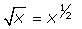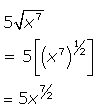Question from Shelia, a student: Write in exponential form 2 sq. root a with an exponent 5.   I do not understand on how to do it. Hi Sheila. The question hinges on your understanding that a square root is equivalent to the exponent one-half:The other important part of the question relies on your knowledge that when you raise a term that has an exponent in it to another exponent, you muliply the exponents. So here's how you would solve a similar question to yours:The last line is in exponent form. Now you try it with your question. Stephen La Rocque.>